## Tuesday, June 30, 2009

### Derivative and Integral of the Heaviside Step Function

The Setup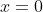the function looks exactly the same.

The Heaviside step function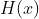, sometimes called the Heaviside theta function, appears in many places in physics, see  for a brief discussion. Simply put, it is a function whose value is zero for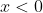and one for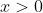. Explicitly,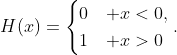(1)
We won't worry about precisely what its value is at zero for now, since it won't effect our discussion, see  for a lengthier discussion. Fig. 1 plots. The key point is that crossing zero flips the function from 0 to 1.

Derivative -- The Dirac Delta Function
Say we wanted to take the derivative of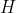. Recall that a derivative is the slope of the curve at at point. One way of formulating this is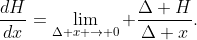(2)
Now, for any pointsor, graphically, the derivative is very clear:is a flat line in those regions, and the slope of a flat line is zero. In terms of (2),does not change, so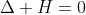and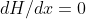. But if we pick two points, equally spaced on opposite sides of, say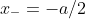and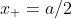, then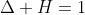and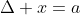. It doesn't matter how small we make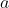,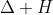stays the same. Thus, the fraction in (2) is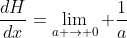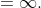(3)
Graphically, again, this is very clear:jumps from 0 to 1 at zero, so it's slope is essentially vertical, i.e. infinite. So basically, we have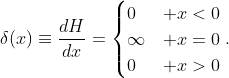(4)
This function is, loosely speaking, a ``Dirac Delta'' function, usually written as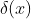, which has seemingly endless uses in physics.

We'll note a few properties of the delta function that we can derive from (4). First, integrating it from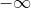to any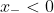: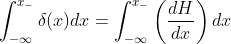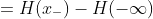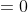(5)
since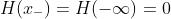. On the other hand, integrating the delta function to any point greater than: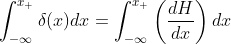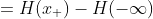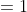(6)
since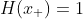.

At this point, I should point out that although the delta function blows up to infinity at, it still has a finite integral. An easy way of seeing how this is possible is shown in Fig. 2(a). If the width of the box is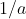and the height is, the area of the box (i.e. its integral) is, no matter how largeis. By lettinggo to infinity we have a box with infinite height, yet, when integrated, has finite area.

Integral -- The Ramp Function
Now that we know about the derivative, it's time to evaluate the integral. I have two methods of doing this. The most straightforward way, which I first saw from Prof. T.H. Boyer, is to integratepiece by piece. The integral of a function is the area under the curve,1 and whenthere is no area, so the integral fromto any point less than zero is zero. On the right side, the integral to a point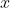is the area of a rectangle of height 1 and length, see Fig. 1(a). So, we have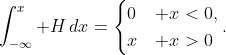(7)
We'll call this function a ``ramp function,''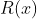. We can actually make use of the definition ofand simplify the notation: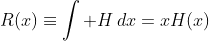(8)
since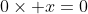and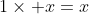. See Fig. 2(b) for a graph -- and the reason for calling this a ``ramp'' function.

But I have another way of doing this which makes use of a trick that's often used by physicists: We can always add zero for free, since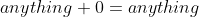. Often we do this by adding and subtracting the same thing,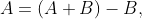(9)
for example. But we can use the delta function (4) to add zero in the form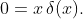(10)
Sinceis zero for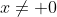, thepart doesn't do anything in those regions and this expression is zero. And, although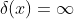at,at, so the expression is still zero.

So we'll add this on to: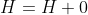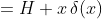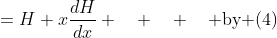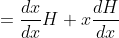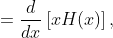(11)
where the last step follows from the ``product rule'' for differentiation. At this point, to take the integral of a full differential is trivial, and we get (8).

References
 M. Springer. Sunday function [online]. February 2009. Available from: http://scienceblogs.com/builtonfacts/2009/02/sunday_function_22.php [cited 30 June 2009].
 E.W. Weisstein. Heaviside step function [online]. Available from: http://mathworld.wolfram.com/HeavisideStepFunction.html [cited 30 June 2009].

1 To be completely precise, it's the (signed) area between the curve and the line.